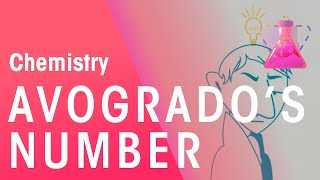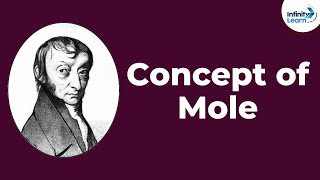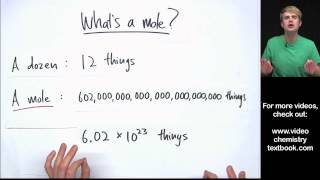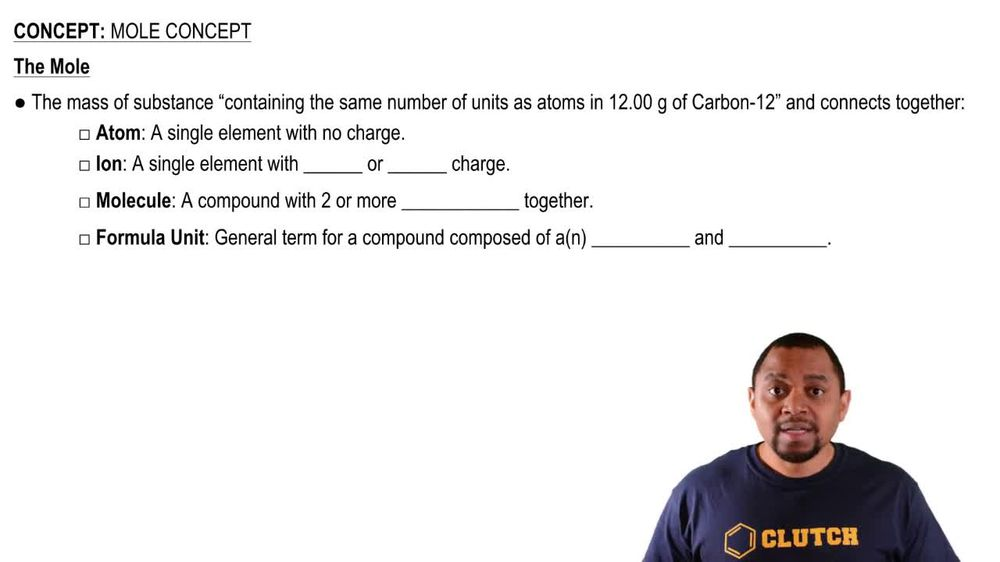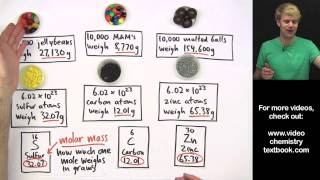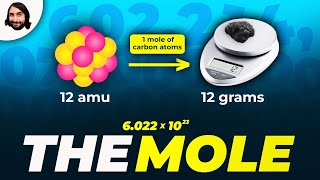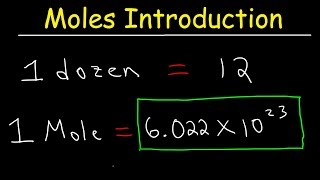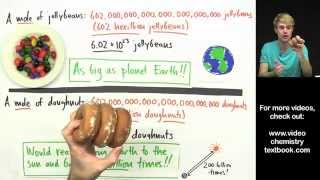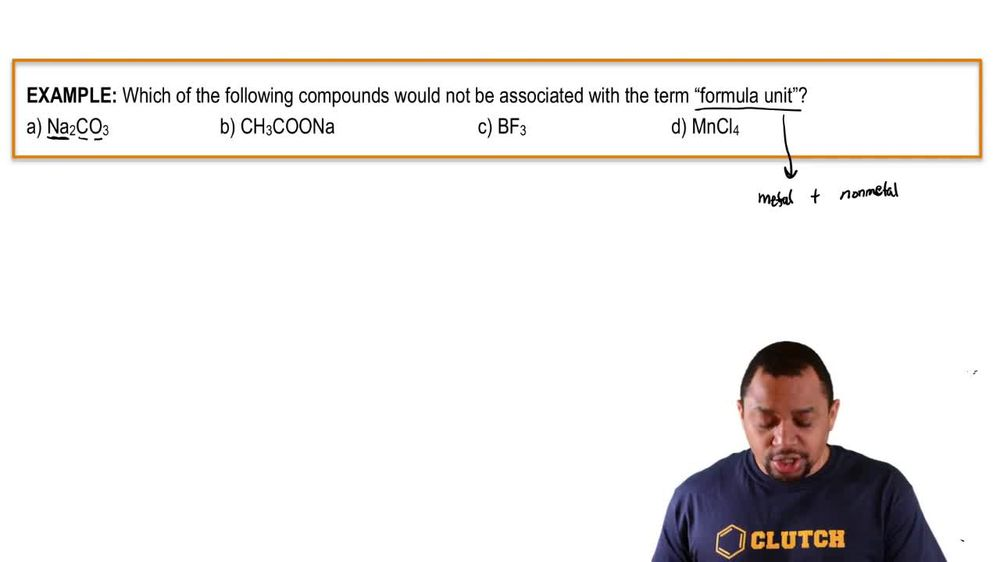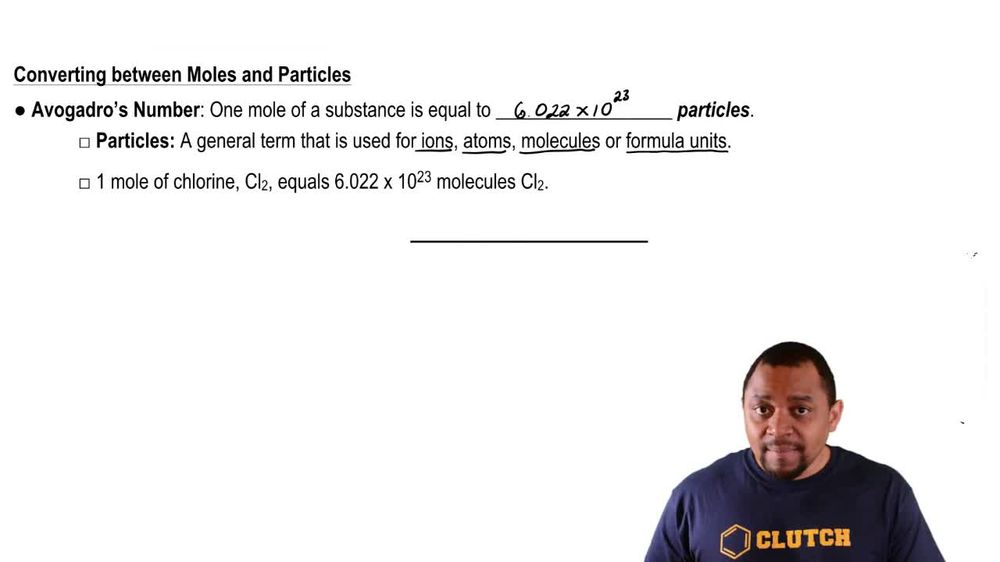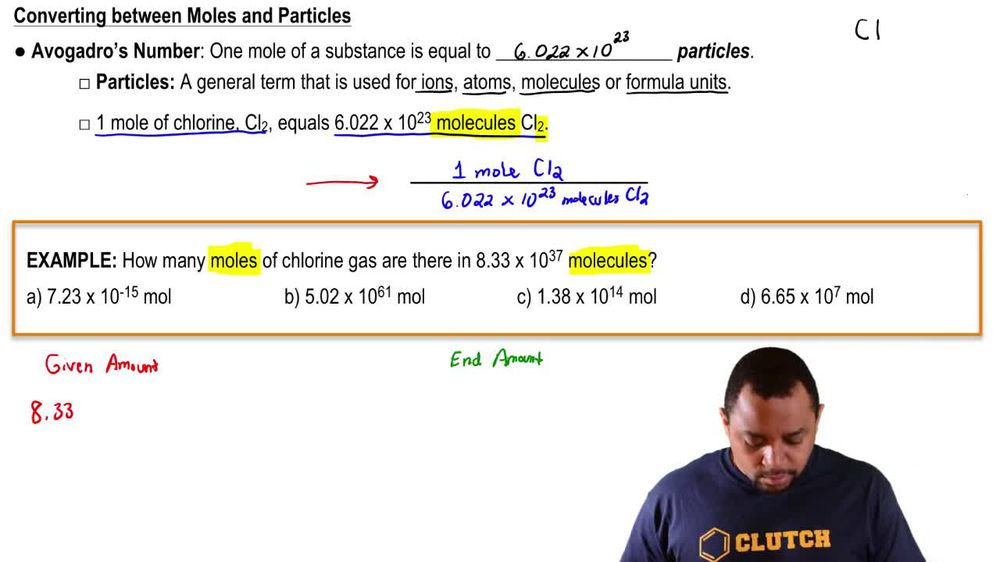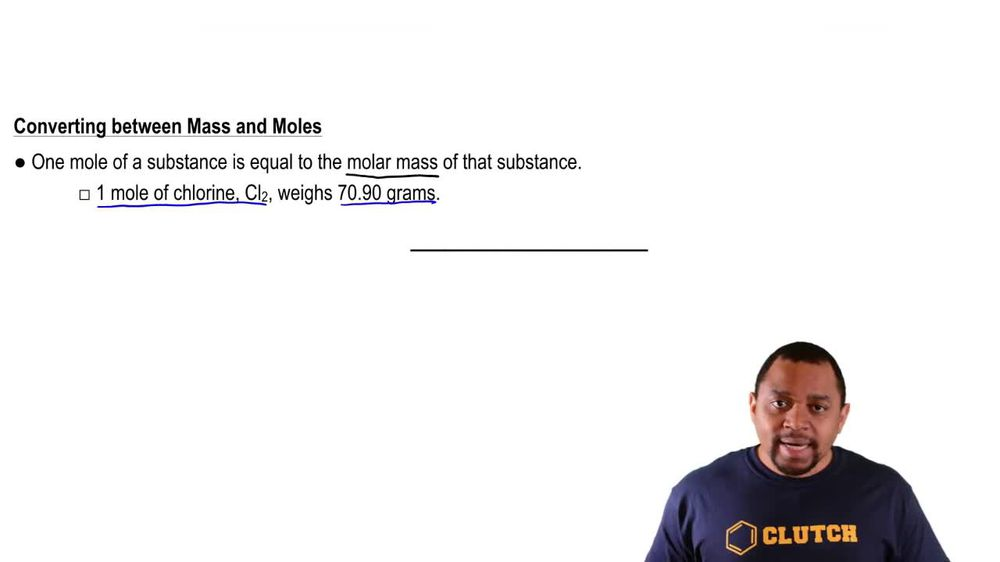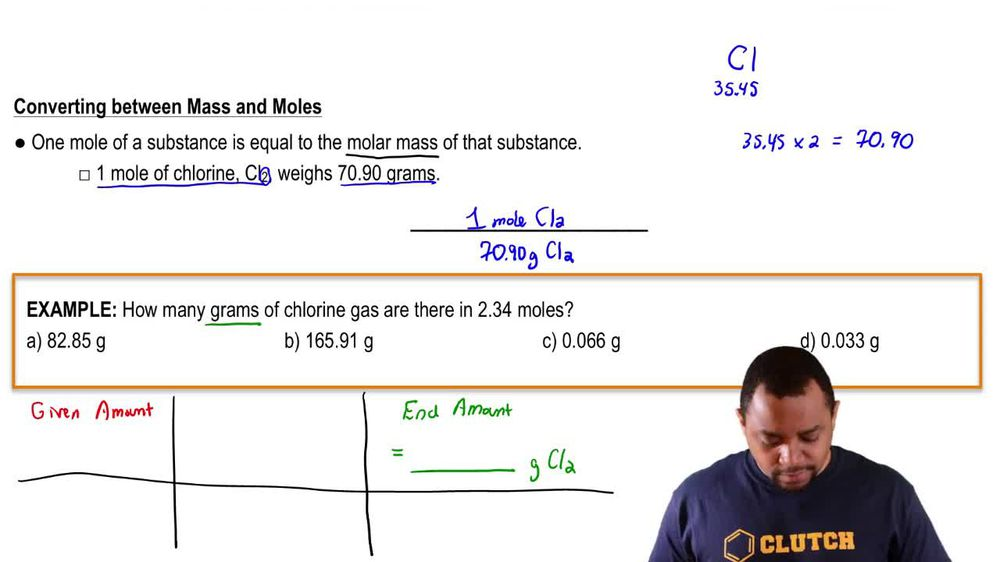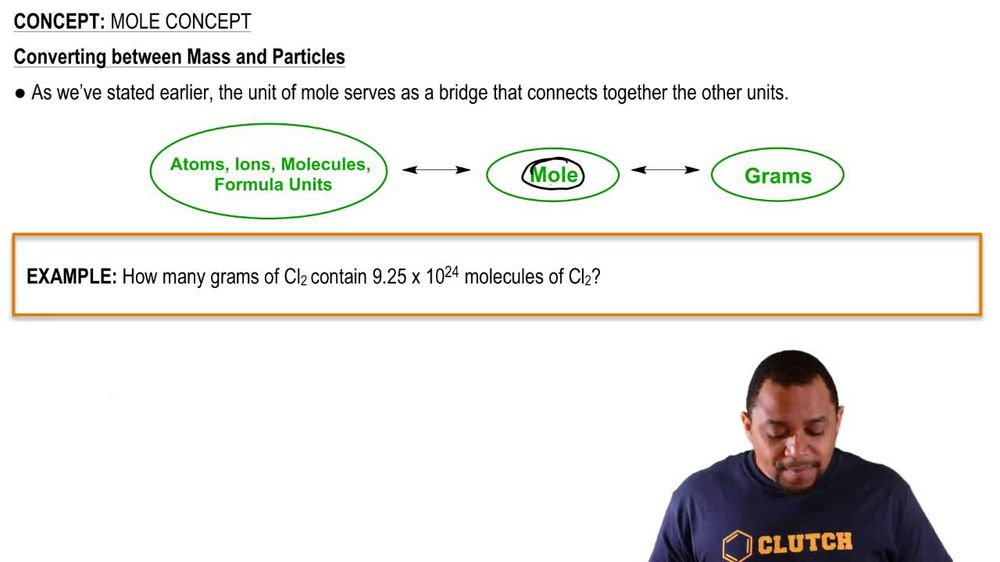Start typing, then use the up and down arrows to select an option from the list.
1. 2. Atoms & Elements2. Mole Concept
Problem

# A pure copper sphere has a radius of 0.935 in. How many copper atoms does it contain? [The volume of a sphere is (4>3)pr3 and the density of copper is 8.96 g/cm3.]

Relevant Solution3m
Play a video:
Welcome back everyone in this example, we are told that a metal cube is made out of pure iron and has a side length of 3.50 centimeters. We're told irons density of 7.874 g per cubic centimeter at room temp. And to calculate the number of iron atoms in this metal cube. So because according to the prompt, we're dealing with a cube here. We want to recall the volume of a cube which we would find is equal to the side length Of the Cube raised to the 3rd power. So luckily they give us our side length in the prompt. And so we would say that our volume of our cube is equal to our side length from the prompt being 3. centimeters. And then this is going to be cubed. And so this is going to give us our volume of our cube equal to a value of 42.875 cubic centimeters. So now that we have this value because we understand that our metal cube is made up of pure iron and we have the volume of the cube. We can understand that. We would say our volume would be made up of these iron atoms. And so we would have 42.875 cubic centimeters of iron atoms making up this cube. But because we want our final unit to be atoms of iron, we need to go through store geometry here. So we want to first focus on canceling out our cubic centimeters of iron. So we would recall that for one cc of iron. This will correspond to the irons density which is given in the prompt as 7.874 g per cubic centimeter. And that is why we have one cc here in the denominator. So this is our grams of iron. And now we're able to cancel out our units of cubic centimeters of iron. And now that we are at grams of iron, we want to focus on going from grams to atoms. So before we can go straight to atoms, we need to get rid of our units of grams of iron. So we're going to utilize the molar mass of iron from the product table where we would see we have 55.845 g of iron equal to one mole of iron. And this allows us to cancel out our units of grams of iron. And now that we are at moles of iron, we can easily get into atoms by recalling avocados number which tells us that we have 6.22 times 10 to the 23rd power atoms of iron equal to one mole of iron. And now we're able to cancel our units of moles of iron leaving us with atoms of iron as our final unit. And so what we would get from our quotient above is a value equal to 3.64 times 10 to the 24th power iron atoms inside the cube. And so this here would be our final answer to complete this example, which corresponds to choice see in our multiple choice. So I hope that everything that I reviewed was clear. If you have any questions, please leave them down below, and I will see everyone in the next practice video.